### Home > INT3 > Chapter 9 > Lesson 9.2.1 > Problem9-99

9-99.
1. 9-99.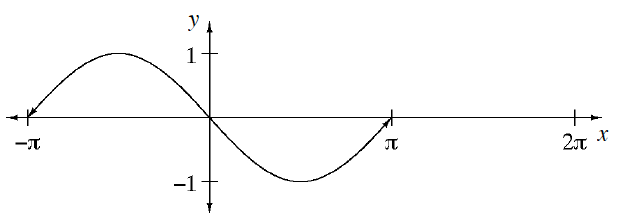The graph at right was made by shifting the first cycle of y = sin(x) to the left. Homework Help ✎

1. How many units to the left was it shifted?

2. Figure out how to change the equation of y = sin(x) so that the graph of the new equation will look like the one in part (a).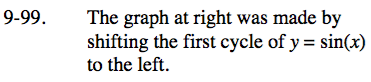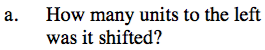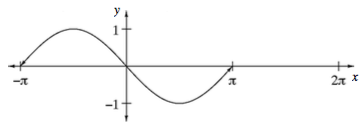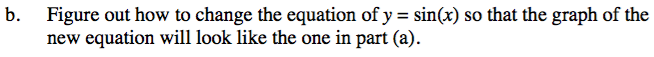In the general equation y = sin(xh) + k, which parameter moves the graph horizontally?